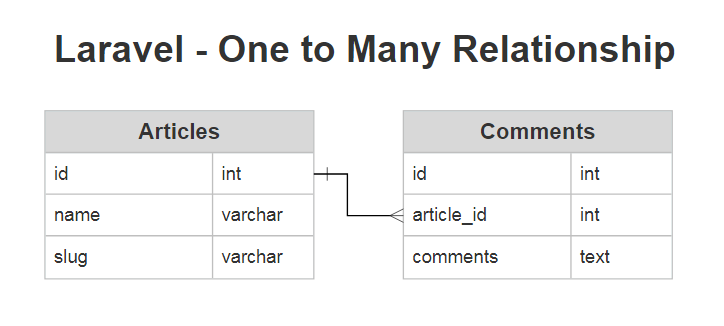# Laravel 8 One To Many Relationship Example

In this article, we will see laravel 8 one to many relationship example. Also, you can use one to many relationship in laravel 6 and laravel 7. A one to many relationships is used to define relationships where a single model is a parent to one or more child models. For one to many relationships use `hasMany and belongsTo` the method in the model for access to each other model. Also, we will create migration with a foreign key, retrieve records using the model, insert new records, sync with a pivot table, etc.

So, let's see one to many relationship in laravel 6/7/8 or laravel 8 hasMany example.

Now, We will create Articles and Comments tables. Connect both tables with each other and create one to many relationships with each other by using the laravel model. To define this relationship, we will place a `comments` functions on the `Article` model. The `comments` function should call the `hasMany` method and return its result.Create Migration

Now, we have to create migration for articles and comments tables. we will also add a foreign key with the articles table.

Create Migration of Articles Table

``````Schema::create('articles', function (Blueprint \$table) {

\$table->increments('id');

\$table->string('name');

\$table->string('slug');

\$table->timestamps();

});``````

Create Migration of Comments Table with Foreign Key

``````Schema::create('comments', function (Blueprint \$table) {

\$table->increments('id');

\$table->integer('article_id')->unsigned();

\$table->timestamps();

});``````

Create Model and Add Relationship on Both Model

In the Article model, we can create the comments() function and add a relation to the Comment model using the hasMany method.

Article Model :

``````<?php

namespace App\Models;

use Illuminate\Database\Eloquent\Model;

class Article extends Model
{
/**
* Get the comments for the article.
*/
{
return \$this->hasMany(Comment::class);
}
}``````

Comment Model:

Now that we can access all of an article's comments, let's define a relationship to allow a comment to access its parent article. To define the inverse of a `hasMany` relationship, define a relationship method on the child model which calls the `belongsTo` method.

``````<?php

namespace App\Models;

use Illuminate\Database\Eloquent\Model;

class Comment extends Model
{
/**
* Get the article that owns the comment.
*/
public function article()
{
return \$this->belongsTo(Post::class);
}
}``````

In the example above, Eloquent will attempt to find a `Article` the model that has an `id` which matches the `article_id` column on the `Comment` model. So, in this example, Eloquent will assume the `Article` model's foreign key on the `comments` table is `article_id`.

if the foreign key on the `Comments` model is not `article_id`, you may pass a custom key name as the second argument to the `belongsTo` method

``````/**
* Get the article that owns the comment.
*/
public function article()
{
return \$this->belongsTo(Article::class, 'foreign_key');
}``````

Retrieve Records using Model :

Once the relationship is defined, we may retrieve the related record using Eloquent's dynamic properties. So, here we can use the Article model with the comments function.

``````\$comments = Article::find(5)->comments;

//
}``````
``````\$comment = Comment::find(7);

\$article = \$comment->article->name;``````

Create Records using Model

Now, we will create records using the comment model.

``````\$article = Article::find(1);

\$comment = new Comment;
\$comment->comments = "One To Many Exmaple";

One To Many Relationship with Query

In all relationships, you can use conditions to query with the model.

``````\$comment = Article::find(1)->comments()
->where('name', 'websolutionstuff')
->first();``````

You might also like: